# Errors, theory of

The branch of mathematical statistics devoted to the inference of accurate conclusions about the numerical values of approximately measured quantities, as well as on the errors in the measurements. Repeated measurements of one and the same constant quantity generally give different results, since every measurement contains a certain error. There are three basic types of error: systematic, gross and random. Systematic errors always either overestimate or underestimate the results of measurements and arise for specific reasons (incorrect set-up of measuring equipment, the effect of environment, etc.), which systematically affect the measurements and alter them in one direction. The estimation of systematic errors is achieved using methods which go beyond the confines of mathematical statistics (see Processing of observations). Gross errors (often called outliers) arise from miscalculations, incorrect reading of the measuring equipment, etc. The results of measurements which contain gross errors differ greatly from other results of measurements and are therefore often easy to identify. Random errors arise from various reasons which have an unforeseen effect on each of the measurements, both in overestimating and in underestimating results.

The theory of errors is only concerned with the study of gross and random errors. The basic problems of the theory of errors are to study the distribution laws of random errors, to seek estimates (see Statistical estimator) of unknown parameters using the results of measurements, to establish the errors in these estimates, and to identify gross errors. Let the values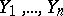be obtained as a result ofindependent, equally accurate measurements of a certain unknown variable. The differences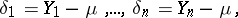are called the true errors. In terms of the probability theory of errors all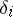are treated as random variables; independence of measurements is understood to be mutual independence of the random variables. The equal accuracy of the measurements is treated broadly as an identical distribution: The true errors of equally accurate measurements are identically distributed random variables. The mathematical expectation of the true errors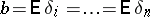is then called the systematic error, while the differences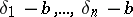are called the random errors. Thus, the absence of a systematic error means that, and, in this situation,are random errors. The variable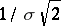, whereis the standard deviation, is called the measure of accuracy (when a systematic error occurs, the measure of accuracy is expressed by the relation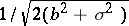). Equal accuracy of measurements is understood in a narrow sense to mean the equality of the measure of accuracy for all the results of the measurements. The incidence of gross errors signifies the disruption of equal accuracy (both in the broad and narrow sense) for certain specific measurements. As an estimator of the unknown valueone usually takes the arithmetic mean from the results of the measurements: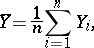while the differencesare called the apparent errors. The choice of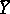as an estimator foris based on the fact that for any sufficiently large numberof equally accurate measurements with no systematic error,, with probability arbitrarily close to one, differs by an arbitrarily small amount from the unknown variable(see Law of large numbers);is free of systematic errors (estimators with this property are called unbiased, cf. also Unbiased estimator), and its variance isExperience has shown that in practice the random errorsare very often subject to almost normal distributions (the reasons for this are revealed in the so-called limit theorems of probability theory). In this case the variablehas an almost normal distribution with mathematical expectationand variance. If the distributions ofare exactly normal, then the variance of every other unbiased estimator for, for example the median (cf. Median (in statistics)), is not less than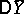. If the distribution ofis not normal, then the latter property need not hold (see the example under Rao–Cramér inequality).

If the varianceof separate measurements is previously unknown, then the variable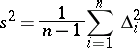is used as estimator for it (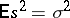, i.e.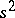is an unbiased estimator for). If the random errorshave a normal distribution, then the relation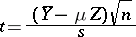is subject to the Student distribution with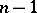degrees of freedom. This can be used to estimate the error of the approximate equality(see Least squares, method of).

The variable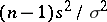has under the same assumptions a "chi-squared" distribution withdegrees of freedom. This enables one to estimate the error of the approximate equality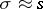. It can be demonstrated that the relative error will not exceed the numberwith probability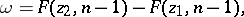where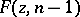is the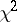-distribution function,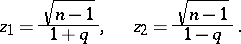If certain measurements contain gross errors, then the above rules for estimatingandwill give distorted results. It is therefore very important to be able to differentiate measurements which contain gross errors from those which are subject only to random errors. For the case whereare independent and have an identical normal distribution, a comprehensive method for identifying measurements which contain gross errors was proposed by N.V. Smirnov .

How to Cite This Entry:
Errors, theory of. Encyclopedia of Mathematics. URL: http://encyclopediaofmath.org/index.php?title=Errors,_theory_of&oldid=28547
This article was adapted from an original article by L.N. Bol'shev (originator), which appeared in Encyclopedia of Mathematics - ISBN 1402006098. See original article